Subsections

# 3.1 Discrete random variables

For the current purposes it is sufficient to think of a discrete random variable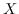as variable with a fixed but unknown integer value larger than or equal to zero. The random variables we will encounter later will be mostly secret keys, messages, and message tags. Our knowledge about the random variable is completely determined by a vector of positive probabilities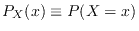, each describing how confident we are that the variable's value is the specific integer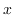, adding up to 1. It is often better to talk about uncertainty, or entropy, instead of knowledge. No uncertainty means full knowledge, i.e., 100% probability for one value and 0% for the rest.

An important special case is the random variables for which all non-zero probabilities are equal. These random variables are called uniform random variables. If Alice throws a normal, but perfect, six-sided die and keeps the result secret, the result is to Bob a uniform random variable with six possible values. If Bob had managed to replace Alice's die with one that is not perfect, the variable would not have been completely uniform. In any case, Alice knows the value so to her it is a random variable with zero uncertainty or entropy.

The range ofis the set of valuescan have, even those with zero probability, and is denoted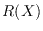. Even though infinite ranges are possible, we will limit ourselves to random variables with finite ranges. Without loss of generality we will only consider ranges consisting of integers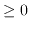.

The expectation value is denoted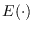and can be defined as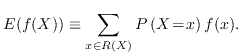(3.1)

Throughout the rest of this thesis the class of random variables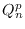defined by (3.2) will serve as an illustrative example. The same definition in Python code is available as function Q in entropies.py line 54 .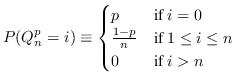(3.2)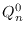is a uniform random variable with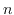possible values. If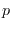is largehas one very probable andequally improbable values. Such random variables are rather extreme and will therefore nicely illustrate some somewhat unintuitive situations later.

# 3.2 Dependent random variables

Two random variablesand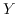can be related in ways that are unrelated to their internal probability vectors. To completely specify both their respective probability vectors and their relations it is sufficient (and necessary) to (be able to) specify the probability vector of a larger random variable, the concatenation of the two variables, written as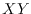, with probabilities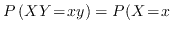and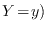. Observe that neithernor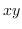are products. When the random variables are related in this way the probabilities in their respective smaller probability vectors are called marginal probabilities.

As an example, consider the dependent random variables defined bywhich can be seen as the two bits of the uniform random variable with values 0, 1 and 2. Their marginal distributions are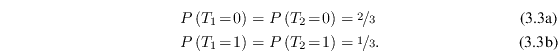Given two random variablesand, when learning that the value ofis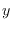the probability vector ofcan change. If they are dependentcontains information aboutand receiving information changes the probabilities. The new random variable can be denoted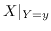and its probability vector is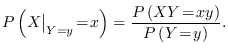(3.5)

This relation is called Bayes' theorem and is a fundamental part of probability theory, but the notation is unorthodox.

Normally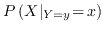is written as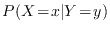and is read as the probability thatequalsgiven thatequals. Similar notations are used for other things, most notably for conditional entropy. We will use the unconventional notation exclusively, both to note explicitly that we are working on a new random variable and to bring the implicit hidden expectation value in conditional entropy written the conventional way out into the light. See Chapter 4.4.1 for more details.

Using Bayes' theorem on the previously defined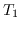and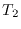yields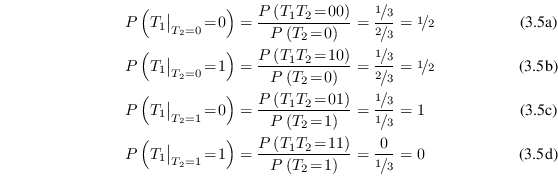and, because of the symmetry in their definition, this also holds whenandare interchanged.

With more than one random variable it can be necessary to specify the expectation value over just one of them. A natural definition is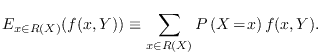(3.7)

# 3.3 Jensen's inequality

There are lots of standard inequalities that are very useful in connection with random variables. We will only need one of them, Jensen's inequality.

Jensen's inequality is applicable to convex and concave functions. A function is called convex if it is continuous and the whole line between every two points in its graph lies on or above the graph. If the whole line lies above the graph it is also called strictly convex. A function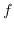is concave3.1 or strictly concave if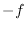is convex or strictly convex. In other words, for all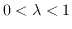,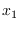, and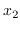holds: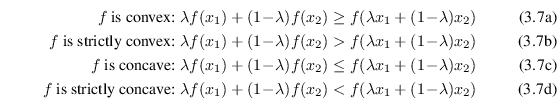Jensen's inequality states that ifis a convex or concave function, then for any random variable: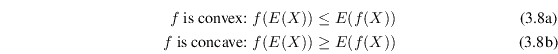Furthermore, ifis strictly convex or strictly concave, equality occurs if and only if there is only one value ofthat is assigned a non-zero probability.

For a random variable with only two possible values, Jensen's inequality just restates the definition of convexity. Generalizing to arbitrary number of values by induction is pretty straightforward and is explained in many other places.

Ifis convex and has an inverse, an alternative way to express Jensen's inequality is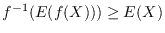.